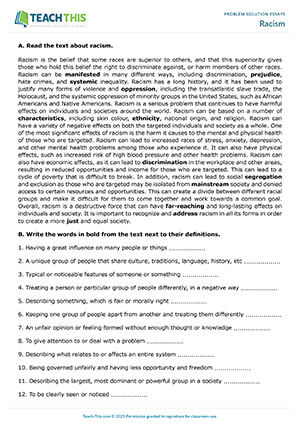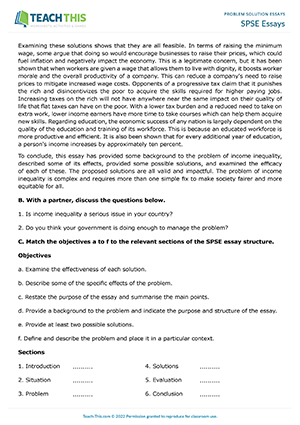# Problem Solution Essays EAP Worksheets

## Problem Solution Essays 1

### EAP Problem Solution Essays Worksheet - Writing Exercises: Gap-fill, Categorising, Identifying, Brainstorming, Listing, Creating an Essay Outline, Writing an Essay - Upper-intermediate (B2) - 90 minutes

In this free problem solution essays worksheet, students are introduced to problem solution essay structure and attempt a problem solution essay writing practice. First, students read a description of problem solution essays and compare two structures for organising a problem solution essay - block structure and chain structure. Students then complete sentences with phrases used for problem solution essays. Next, students arrange the phrases into a table according to their function and add any additional phrases they may know to the table. After that, students read an example problem solution essay and underline language that indicates a problem or a solution. Next, with a partner, students discuss three problems and think of some solutions. Students then choose one problem and create a block structure essay outline on the topic. Following that, students use the outline to write their own problem solution essay. Finally, students swap their essay with another student and do a peer review.## Racism

### EAP Problem Solution Essays Worksheet - Reading and Writing Exercises: Outlining, Gap-fill, Writing an Essay - Speaking Activity: Discussion, Communicative Practice - Upper-intermediate (B2) - 90 minutes

In this handy problem solution essay writing practice, students read and discuss a text on the issue of racism and then plan and write a problem solution essay that aims to address the problem. First, students read a text about racism and then match words from the text to their definitions. Next, students discuss the problems mentioned in the text that are linked to racism and come up with some potential solutions. Students then use information from the text and their ideas to prepare a problem solution essay outline that addresses the issue. After that, students complete some advice about problem solution essay writing with the words provided. Next, students use their outline to write a problem solution essay that addresses the issue of racism. Finally, students swap papers with a partner and use a checklist to review and provide feedback on their partner's essay.## SPSE Essays

### EAP Problem Solution Essays Worksheet - Writing: Matching, Writing an Essay - Speaking Activity: Guided Discussion, Communicative Practice - Group Work - Upper-intermediate (B2) - 90 minutes

In this productive SPSE essays worksheet, students are introduced to SPSE essay structure and attempt an SPSE essay writing practice. First, students read a short description of SPSE essays and an example essay on the topic of income inequality. In pairs, students then discuss two income inequality questions. Next, students match a list of objectives to the relevant sections of the SPSE essay structure. Students then move on to read six example sentences from an SPSE essay and match them to the relevant section of the SPSE structure. Following that, in groups, students choose one of the problems listed and discuss the current situation and related sub-problems. Students then discuss some possible solutions and evaluate each of the solutions by examining their respective pros and cons, making notes in the table provided. After that, students use the notes to create an SPSE essay outline. Students then read a short checklist to help them write their SPSE essay. Afterwards, students write a practice SPSE essay using their outline and checklist.Now Available!